# Google Kick Start Archive — Round E 2021 problems

## Overview

Thank you for participating in Kick Start 2021 Round E!

Cast

Shuffled Anagrams: Written by Pablo Heiber and prepared by Swapnil Mahajan.

Birthday Cake: Written by Mohamed Omar and prepared by Laksh Nachiappan.

Palindromic Crossword: Written by Bartosz Kostka and prepared by Attila Toth.

Increasing Sequence Card Game: Written by Yossi Matsumoto and prepared by Swapnil Gupta.

Solutions, other problem preparation, reviews and contest monitoring by Abhishek Saini, Abhishek Singh, Akul Siddalingaswamy, Alexandra Goodman, Aneesh D H, Anik Sarker, Ankit Goyal, Anurag Singh, Anushi Maheshwari, Attila Toth, Bartosz Kostka, Bohdan Pryshchenko, Erick Wong, Gregory Yap, Harshil Shah, Jared Gillespie, Jennifer Zhou, Kashish Bansal, Krists Boitmanis, Laksh Nachiappan, Lizzie Sapiro Santor, Lucas Maciel, Mahmoud Ezzat, Manav Jain, Mohamed Omar, Pablo Heiber, Pranjal Jain, Rudhir Gupta, Ruoyu Zhang, Samiksha Gupta, Sarah Young, Sasha Fedorova, Sera Wang, Sharath Holla, Shweta Karwa, Swapnil Gupta, Swapnil Mahajan, Swetank Modi, Szymon Rzeźnik, Teja Vardhan Reddy Dasannagari, Umang Goel, Utsav Rajpara, Vijay Krishan Pandey, Wajeb Saab, Wei Zhou, Yossi Matsumoto.

Analysis authors:

• Shuffled Anagrams: Sarah Young
• Birthday Cake: Krists Boitmanis
• Palindromic Crossword: Ankit Goyal
• Increasing Sequence Card Game: Ankit Goyal

## A. Shuffled Anagrams

Let $$\mathbf{S}$$$be a string containing only letters of the English alphabet. An anagram of $$\mathbf{S}$$$ is any string that contains exactly the same letters as $$\mathbf{S}$$$(with the same number of occurrences for each letter), but in a different order. For example, the word kick has anagrams such as kcik and ckki. Now, let $$S[i]$$$ be the $$i$$$-th letter in $$\mathbf{S}$$$. We say that an anagram of $$\mathbf{S}$$$, A, is shuffled if and only if for all $$i$$$, $$S[i] \neq A[i]$$$. So, for instance, kcik is not a shuffled anagram of kick as the first and fourth letters of both of them are the same. However, ckki would be considered a shuffled anagram of kick, as would ikkc. Given an arbitrary string $$\mathbf{S}$$$, your task is to output any one shuffled anagram of $$\mathbf{S}$$$, or else print IMPOSSIBLE if this cannot be done. ### Input The first line of the input gives the number of test cases, $$\mathbf{T}$$$. $$\mathbf{T}$$$test cases follow. Each test case consists of one line, a string of English letters. ### Output For each test case, output one line containing Case #$$x$$$: $$y$$$, where $$x$$$ is the test case number (starting from 1) and $$y$$$is a shuffled anagram of the string for that test case, or IMPOSSIBLE if no shuffled anagram exists for that string. ### Limits Memory limit: 1 GB. $$1 \le \mathbf{T} \le 100$$$.
All input letters are lowercase English letters.

#### Test Set 1

Time limit: 20 seconds.

### Sample

Sample Input
2
start
jjj

Sample Output
Case #1: tarts
Case #2: IMPOSSIBLE


In test case #1, tarts is a shuffled anagram of start as none of the letters in each position of both strings match the other. Another possible solution is trsta (though you only need to provide one solution). However, in test case #2, there is no way of anagramming jjj to form a shuffled anagram, so IMPOSSIBLE is printed instead.

## B. Birthday Cake

### Problem

You are given a grid of $$\mathbf{R}$$$rows and $$\mathbf{C}$$$ columns that corresponds to a birthday cake.
The rows are numbered from $$1$$$to $$\mathbf{R}$$$ starting from the top. The columns are numbered from $$1$$$to $$\mathbf{C}$$$ starting from the left. Each cell in the grid is a square of size $$1 \times 1$$$. You noticed that the most delicious part of the cake forms a single filled rectangle; that means all the cells inside this single rectangle will be delicious as well, but all the cells outside this rectangle are not delicious. You have a knife that is long enough to make straight-line cuts of length up to $$\mathbf{K}$$$.

We want to make a series of cuts to extract each of the delicious cells separately, so that we can put candles on them, and enjoy the birthday party.
To extract each of the delicious cells separately, they must be disconnected from any other cell.
A cell is disconnected if no other cell is connected to it in any of the $$4$$$directions (up, down, left, right). A cut is a directed line segment which is valid if the following conditions are met: • The cut runs along one of the horizontal or vertical lines between the rows and columns of the grid. • The length of the cut must not exceed $$\mathbf{K}$$$.
• The starting and ending points of the cut must be grid points (i.e. a corner of a cell). In addition, the starting point must be already exposed, meaning that it lies on one of the $$4$$$sides of the grid or on one of the previous cuts. • The cut must not pass through any other exposed points. It may touch an exposed point, but if it does, it must end right there. Suppose that $$\mathbf{K} = 4$$$. Below you can find five examples of valid cuts.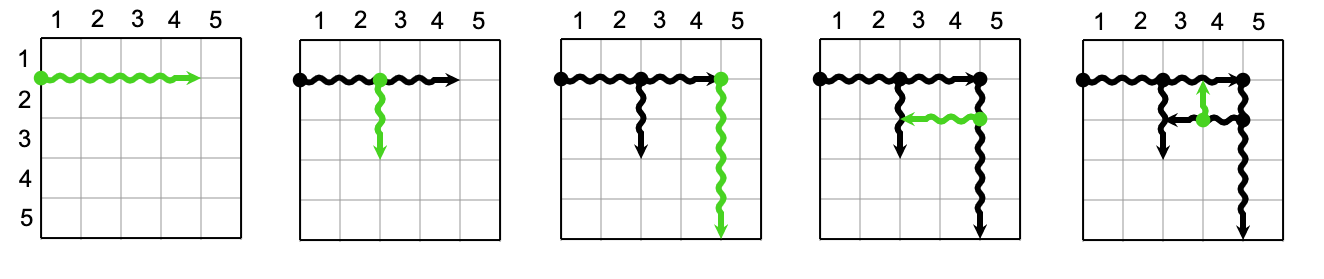And here are four examples of invalid cuts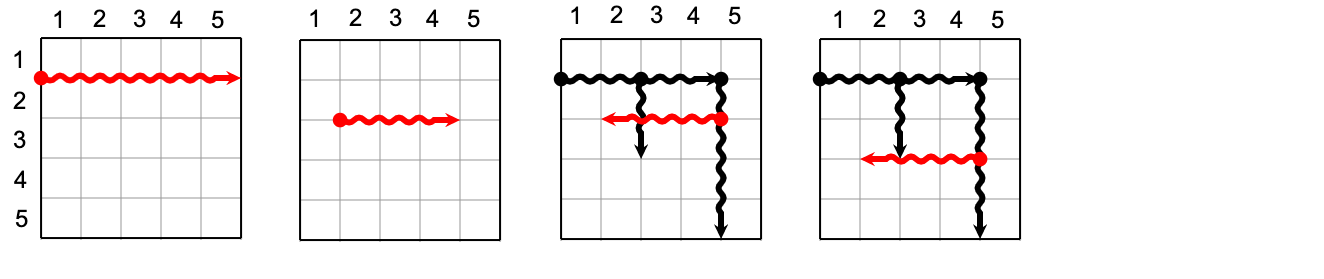• In the first picture, the cut is too long (longer than $$4$$$). • In the second picture, the cut starts from an unexposed point (neither one of the $$4$$$ sides of the grid nor a previous cut).
• In the third picture, the cut passes through an exposed point, it must stop once it touches the exposed point at length $$2$$$. • The fourth picture is invalid because of the same reason as the third picture. We need to find the minimum number of cuts needed to extract all the delicious cells. ### Input The first line of the input gives the number of test cases, $$\mathbf{T}$$$. $$\mathbf{T}$$$test cases follow. Each test case starts with a line containing three integers, $$\mathbf{R}$$$, $$\mathbf{C}$$$and $$\mathbf{K}$$$.
The next line contains four integers, $$\mathbf{r_1}$$$, $$\mathbf{c_1}$$$, $$\mathbf{r_2}$$$, $$\mathbf{c_2}$$$, representing the top-left and bottom-right cell of the delicious rectangle respectively.

### Output

For each test case, output one line containing Case #$$x$$$: $$y$$$, where $$x$$$is the test case number (starting from 1) and $$y$$$ is the minimum number of cuts.

### Limits

Time limit: 10 seconds.
Memory limit: 1 GB.
$$1 \le \mathbf{T} \le 100$$$. $$1 \le \mathbf{r_1} \le \mathbf{r_2} \le \mathbf{R}$$$.
$$1 \le \mathbf{K} \le 10^5$$$. ### Sample Note: there are additional samples that are not run on submissions down below. Sample Input 1 3 3 1 2 2 2 2  Sample Output Case #1: 5  ### Additional Sample - Test Set 2 The following additional sample fits the limits of Test Set 2. It will not be run against your submitted solutions. Sample Input 1 2 3 4 2 1 2 2  Sample Output Case #1: 3  In the Sample Case, the minimum number of cuts is $$5$$$. One of the possible series of cuts is as follows: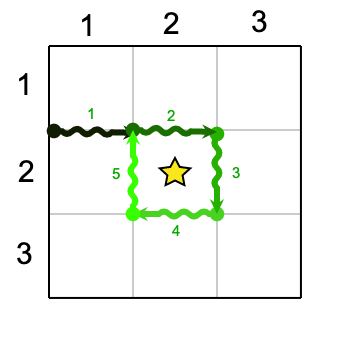In the Additional Sample Case, the minimum number of cuts is $$3$$$. One of the possible series of cuts is as follows: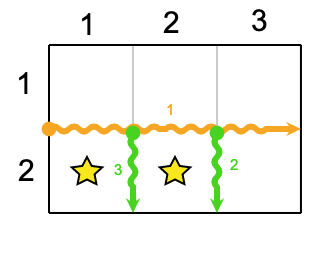## C. Palindromic Crossword ### Problem A crossword puzzle is a rectangular grid of black cells and letters A-Z like the one shown below.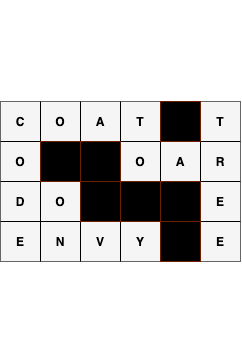Words in the crossword are defined as maximal vertical or horizontal segments of characters. In the crossword below, DO and ON are examples of words.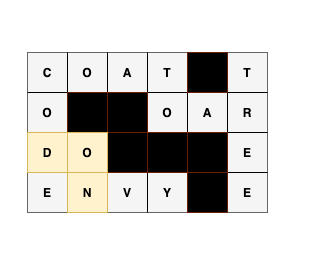A palindromic crossword is one where every word is a palindrome. Let $$\mathbf{R_{i,j}}$$$ represent the character on the $$i$$$-th row and $$j$$$-th column, where $$i$$$and $$j$$$ are $$1$$$-indexed. The top left corner is $$\mathbf{R_{1,1}}$$$. In the example palindromic crossword below, the B in $$\mathbf{R_{3,2}}$$$is part of both the horizontal word starting at $$\mathbf{R_{3,1}}$$$ and the vertical word ending at $$\mathbf{R_{4,2}}$$$, and both are palindromes.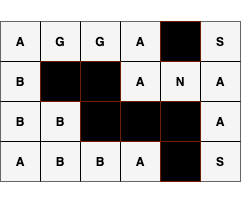You have been gifted a palindromic crossword puzzle with $$\mathbf{N}$$$ rows and $$\mathbf{M}$$$columns. You finished the crossword and throw away the clues, preparing to hang it on your wall. However, you accidentally erase some of the letters! You want to recover as much of the crossword as possible, but you do not have the clues anymore. Using only the knowledge that the crossword is palindromic, restore the maximum possible number of missing characters in the given crossword. Missing letters are represented as empty white cells in the below diagram. The crossword on the left is the crossword you are given and the crossword on the right is the result after you recover as many letters as possible. The remaining cells cannot be filled because we do not have sufficient information to recover them.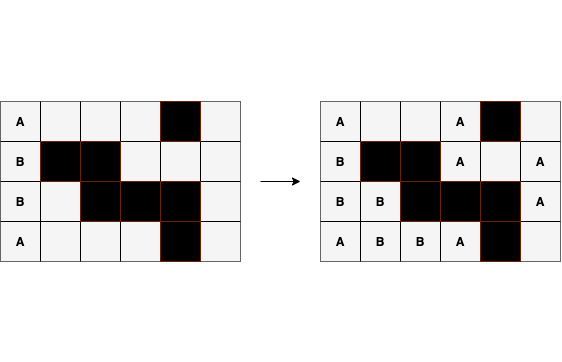### Input The first line of the input gives the number of test cases, $$\mathbf{T}$$$. $$\mathbf{T}$$$test cases follow. The first line of each test case contains two integers, $$\mathbf{N}$$$ and $$\mathbf{M}$$$, representing the number of rows and columns in the crossword, respectively. The next $$\mathbf{N}$$$ lines represent the $$\mathbf{N}$$$rows of the grid. The $$i$$$-th row consists of $$\mathbf{M}$$$characters representing $$\mathbf{R_{i,1}}$$$, $$\mathbf{R_{i,2}}$$$, $$\dots$$$, $$\mathbf{R_{i,M}}$$$. Each character is one of the following: • A capital letter of the alphabet (A-Z) • A period (.) for a missing letter (empty white cell in the example crossword) • A hash (#) for black cell ### Output For each test case, output one line containing Case #$$x$$$: $$y$$$ where $$x$$$ is the test case number (starting from $$1$$$) and $$y$$$ is the number of empty white cells that were filled. Then, output $$\mathbf{N}$$$more lines representing the final grid, with the missing characters (.) replaced by capital letters (A-Z) where possible. ### Limits Time limit: 60 seconds. Memory limit: 1 GB. $$1 \le \mathbf{T} \le 100$$$.
There exists at least one way to fill in the given input grid such that it is a palindromic crossword.
All characters in the grid are in the set $$\{$$$A-Z, #, .$$\}$$$

$$1 \le \mathbf{N},\mathbf{M} \le 50$$$. #### Test Set 2 For at most 10 cases: $$1 \le \mathbf{N},\mathbf{M} \le 1000$$$.

For the remaining cases:
$$1 \le \mathbf{N},\mathbf{M} \le 50$$$. ### Sample Sample Input 2 2 2 A. .# 4 6 A...#. B##... B.###. A...#.  Sample Output Case #1: 2 AA A# Case #2: 8 A..A#. B##A.A BB###A ABBA#. In Sample Case #2, we are able to fill in $$8$$$ of the blanks. We can fill in the missing letters as follows:

• row $$1$$$, column $$4$$$: We know this is A from character at row $$1$$$, column $$1$$$.
• row $$2$$$, column $$4 =$$$ A from row $$1$$$, column $$4$$$.
• row $$2$$$, column $$6 =$$$ A from row $$2$$$, column $$4$$$.
• row $$3$$$, column $$6 =$$$ A from row $$2$$$, column $$6$$$.
• row $$3$$$, column $$2 =$$$ B from row $$3$$$, column $$1$$$.
• row $$4$$$, column $$2 =$$$ B from row $$3$$$, column $$2$$$.
• row $$4$$$, column $$3 =$$$ B from row $$4$$$, column $$2$$$.
• row $$4$$$, column $$4 =$$$ A from row $$4$$$, column $$1$$$.
• ## D. Increasing Sequence Card Game

### Problem

You're playing a card game as a single player.

There are $$\mathbf{N}$$$cards. The $$i$$$-th card has integer $$i$$$written on it. You first shuffle $$\mathbf{N}$$$ cards randomly and put them in a pile. Take the card at the top of the pile to your hand. Then repeat the following process until the pile becomes empty:

1. Check the card on the top of the pile.
2. If the number on the card is larger than the number on the last card you took, take the card.

### Output

For each test case, output one line containing Case #x: y, where x is the test case number (starting from 1) and y is the expected score at the end of the game.

y will be considered correct if it is within an absolute or relative error of 10-6 of the correct answer. See the FAQ for an explanation of what that means, and what formats of real numbers we accept.

### Limits

Time limit: 20 seconds.
Memory limit: 1 GB.

### Sample

Sample Input
2
1
2

Sample Output
Case #1: 1.0
Case #2: 1.5


## Analysis — A. Shuffled Anagrams

### Test set 1

For this test set, since the length of $$\mathbf{S}$$$$$\le 8$$$, we can try every permutation of characters and check whether there exists a permutation such that for all $$i$$$, $$S[i] \neq A[i]$$$. To find every permutation, we can first convert the string to a character array. Then, we swap the first element with every other element and recursively find permutations of the rest of the string.

This can be performed in $$O(N!)$$$, where $$N$$$ is the length of $$\mathbf{S}$$$. ### Test set 2 For this test set, the above solution would exceed the time limits. The key observation here is that if a character exists more than $$\lfloor \frac{N}{2} \rfloor$$$ times, then it's impossible to find such a permutation, because at least one position will have a letter that stays the same. Otherwise, we can sort the letters and keep track of the initial position of each letter.

Let the new sorted letters be $$P$$$. We can split the sorted letters into two halves, from index $$0$$$ to $$\frac{N}{2}$$$, $$P[0:\frac{N}{2}]$$$, and from $$\frac{N}{2}$$$to the end, $$P[\frac{N}{2}:]$$$. If $$N$$$is odd, split $$P$$$ such that the second half has an extra letter, where the first half is $$0$$$to $$\lfloor \frac{N}{2} \rfloor$$$ and the second half is from $$\lceil \frac{N}{2} \rceil$$$to the end. Then, we put each character from the second half of the sorted letters $$P[i + (\frac{N}{2})]$$$ into the original position of the corresponding letter in the first half $$P[i]$$$. Similarly, we put each character from the first half of the sorted letters $$P[i]$$$ into the original position of the corresponding letter in the second half $$P[i + (\frac{N}{2})]$$$. Note that if $$N$$$ is odd, the second half of the sorted letters $$P[i + (\frac{N}{2})]$$$will occupy the first $$\lfloor \frac{N}{2} \rfloor +1$$$ spaces, while the original first half will occupy the last $$\lfloor \frac{N}{2} \rfloor$$$spaces, as shown in the example below. The letter orginally at $$P[N-1]$$$ will be in the middle of the array after the swap, replacing $$P[i + \lfloor \frac{N}{2} \rfloor]$$$.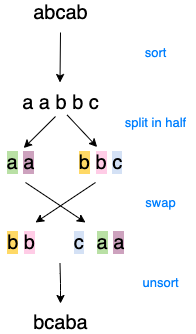This works because we know that no more than half the characters are equal, and hence the character at $$P[i]$$$ cannot be equal to the letter at $$P[i + (\frac{N}{2})]$$$. This can be performed in $$O(N \log N)$$$, due to sorting. However, due to the limited size of the alphabet, we can actually sort even faster using a non-comparative sorting algorithm such as counting sort.

Test Data
info We recommend that you practice debugging solutions without looking at the test data.

## Analysis — B. Birthday Cake

In what follows, let us suppose that the delicious part of the cake has $$n$$$rows and $$m$$$ columns.

### Test Set 1

When $$\mathbf{K}=1$$$, we must consider two cases. If the delicious part is fully inside the cake, meaning that the outer cells are not delicious, then all grid lines around the delicious cells must be cut. Specifically, there are $$n+1$$$ grid lines of length $$m$$$and $$m+1$$$ grid lines of length $$n$$$, so the answer is $$n(m+1)+m(n+1)=2nm+n+m$$$ plus the minimum distance to cut from the border to the delicious part. On the other hand, if the delicious part shares some border with the whole cake, then the answer is $$2nm+n+m$$$minus the length of the shared border. The time complexity is $$O(1)$$$.

### Test Set 2

Let us start out simple. Suppose a cake of size $$n \times m$$$is entirely delicious and needs to be cut into $$1 \times 1$$$ squares. This means that we need to cut the cake along all internal grid lines, which are marked bold in the following picture. Moreover, suppose for the moment that our knife is infinitely long. One way to cut the cake would be to make $$n-1$$$horizontal cuts and then make $$m-1$$$ vertical cuts for each of the resulting $$n$$$horizontal strips. This strategy amounts to $$n-1+n(m-1)=nm-1$$$ cuts. If we start with $$m-1$$$long vertical cuts first, then we would need $$n-1$$$ horizontal cuts for each of the $$m$$$vertical strips, which again results in $$m-1+m(n-1)=nm-1$$$ cuts.Can we do any better? No. In order to prove this claim, let us consider the worst case scenario where we make cuts of length $$1$$$only, which means $$n(m-1)+m(n-1)=2nm-n-m$$$ cuts in total. Since we have a very long knife, we can try to combine or merge some of those unit cuts into longer cuts. Now, at each internal grid point, we can merge the two vertical cuts meeting at that point or the two horizontal cuts, but not both. Thus, we can save at most one cut at each internal grid point. There are $$(n-1)(m-1)=nm-n-m+1$$$internal grid points, which is how many cuts we can possibly save. It follows that we need at least $$2nm-n-m-(nm-n-m+1)=nm-1$$$ cuts to divide the cake into unit squares.

Now that we know how to cut a fully delicious cake with a sufficiently long knife (i.e. $$\mathbf{K} \ge n$$$or $$\mathbf{K} \ge m$$$), let us turn our attention to the case where the length of the knife is $$\mathbf{K} \lt \min(n,m)$$$. With a cake this large, some cuts will necessarily terminate at a previously unexposed internal grid point. For convenience, we will call them red points. And if we continue the reasoning from the previous paragraph, the red points will not save a cut for us. In other words, as soon as we stop at a previously unexposed grid point, the three other grid lines meeting at that point will belong to different cuts with no chance of merging any two of them. Conversely, if a cut exposes an internal grid point without terminating at it, then that grid point belongs to just three different cuts and so one cut is saved. Consequently, we want to minimize the number of red points. To prove a lower bound on the number of red points, let us consider $$\lfloor \frac{n-1}{\mathbf{K}} \rfloor$$$ rows of $$\mathbf{K} \times \mathbf{K}$$$blocks, $$\lfloor \frac{m-1}{\mathbf{K}} \rfloor$$$ blocks in each row as shown in the following picture for $$\mathbf{K}=3$$$. If we consider the blocks open at the left and bottom sides and closed at the right and top sides, then each of the blocks contains $$\mathbf{K} \times \mathbf{K}$$$ internal grid points and any two blocks are disjoint. One can easily verify that the very first cut that has a point common with a particular block will create a red point in that block. Since the blocks are disjoint, there will be at least $$\lfloor \frac{n-1}{\mathbf{K}} \rfloor \times \lfloor \frac{m-1}{\mathbf{K}} \rfloor$$$red points for any cutting strategy.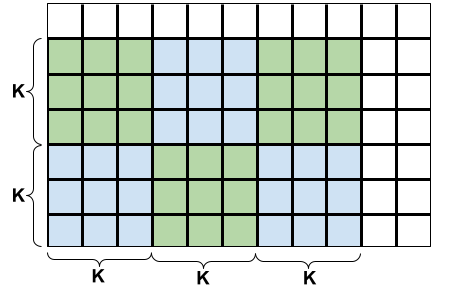It turns out that for an optimal cutting strategy this is also the upper bound on the number of red points. One way of achieving this bound is to select the top-right corner of each block as the red point, cut out the blocks with $$2\lfloor \frac{n-1}{\mathbf{K}} \rfloor \times \lfloor \frac{m-1}{\mathbf{K}} \rfloor$$$ full cuts, and finally cut all the blocks and the L-shaped remaining part into unit squares without ever terminating at a previously unexposed grid point (i.e. without introducing new red points). Recall that every internal grid point that is not red saves us one cut. It follows that the minimum number of cuts to partition a fully delicious cake is $$nm-1+\lfloor \frac{n-1}{\mathbf{K}} \rfloor \times \lfloor \frac{m-1}{\mathbf{K}} \rfloor$$$. Note that the case with a sufficiently long knife is a special case of this formula, as $$\lfloor \frac{n-1}{\mathbf{K}} \rfloor \times \lfloor \frac{m-1}{\mathbf{K}} \rfloor=0$$$.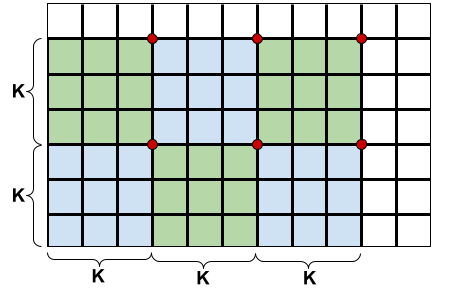It remains to solve the problem for a cake that is partially not delicious. Intuitively, it seems reasonable to cut out the delicious rectangle first and then proceed with the above strategy to partition the delicious part into unit squares. To cut out the delicious rectangle, we should start cutting from the outer border of the cake towards one of the corners of the delicious rectangle and then cut around the rectangle as shown in the following picture for $$\mathbf{K}=3$$$. There are eight symmetric variants to try and we take the minimum number of cuts obtained this way. We should be careful, though, not to include any sides of the delicious rectangle that are touching the border of the whole cake.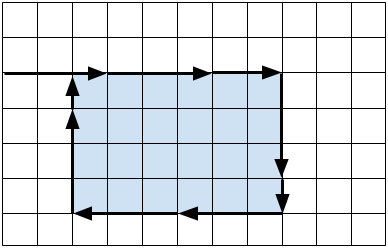A skeptical reader might ask whether it could be worth considering a cutting strategy where we start from the outer border and cut towards somewhere in the middle of the delicious rectangle. If the distance from the border to the delicious rectangle is not divisible by $$\mathbf{K}$$$, then the last step would cut right into the delicious part and perhaps save one cut for the internal grid lines of the delicious rectangle. It can be shown, however, that we would need one extra cut for the outline in this case, so overall we would not gain anything. The proof of this claim is technical yet not very interesting, so it is left for the reader as an exercise.

$$O((\mathbf{N} \times \mathbf{M}) \times (\mathbf{N} \times \mathbf{M})) = O(\mathbf{N}^2 \times \mathbf{M}^2)$$$### Test Set 2 Consider the following crossword: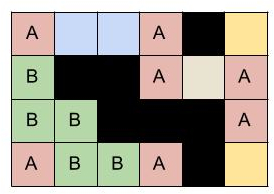This image shows the equivalence classes on a partially solved crossword. Now, if we find these equivalence classes, we can fill all the cells for each class if any of the cells in that class contains a character. Now, to build equivalence classes, let us start by building a graph from our crossword. We will consider all cells in the crossword (which are not '#') as nodes of our graph and draw edges between palindromic positions. To generate these edges, we can traverse over all rows and then over all columns of the crossword. For each row/column, split it into its constituent words and add an edge between the palindromic positions. Now, if two cells belong to the same connected component, they are equivalent. We can find connected components (equivalence classes) optimally from our constructed graph using any graph search algorithm (such as DFS or BFS) or using DSU. Runtime analysis: Any graph traversal algorithm will visit each cell only once and a cell can be connected to at most two other cells. Then, each cell will be visited at most once more when filling in equivalence classes. So, total runtime will be: $$O(\mathbf{N} \times \mathbf{M})$$$

Test Data
info We recommend that you practice debugging solutions without looking at the test data.

## Analysis — D. Increasing Sequence Card Game

### Test Set 1

For the first test set, we can generate all possible arrangements of the cards from $$1$$$to $$\mathbf{N}$$$. For each arrangement of the cards, we can play the game to find the score for that particular arrangement. Then the expected score of the game will be the average score over all arrangements. The total number of possible arrangements for $$\mathbf{N}$$$cards is $$\mathbf{N}!$$$.

The time complexity is $$O(\mathbf{N} \cdot \mathbf{N}!)$$$which is under the required limit for $$\mathbf{N} = 10$$$.

### Test Set 2

Our previous strategy would not work for this test set.
Let us consider arrangements where the number on top of the pile is $$x$$$. Then among the remaining $$\mathbf{N} - 1$$$ cards, all the cards that are smaller than $$x$$$will be discarded. We only need to take care of the ones that are greater than $$x$$$. So, the expected score for arrangements with $$x$$$on top of the pile will be one higher than the expected score of the rest of the cards greater than $$x$$$. Also, it does not matter what the starting and ending number on the cards is, all that matters is the number of cards. So, the expected score of cards from $$x + 1$$$to $$\mathbf{N}$$$ is equal to expected score of cards from $$1$$$to $$\mathbf{N} - x$$$. Assuming $$E_\mathbf{N}$$$denotes the expected score of $$\mathbf{N}$$$ cards, the expected score when $$x$$$is on top is $$E_{\mathbf{N} - x} + 1$$$.

Now, $$x$$$ranges from $$1$$$ to $$\mathbf{N}$$$, so the expected score for $$\mathbf{N}$$$ cards is $$E_\mathbf{N} = \frac{\sum_{x=1}^{\mathbf{N}}(E_{\mathbf{N} - x} + 1)}{\mathbf{N}} = \frac{\sum_{i=0}^{\mathbf{N}-1}E_{i}}{\mathbf{N}} + 1$$$. The summation in the term is a cumulative sum of expected scores of first $$\mathbf{N}-1$$$ natural numbers. So, expected score for $$\mathbf{N}$$$cards can be computed in linear time if we maintain the cumulative sum of the expected score for $$\mathbf{N}-1$$$ cards.

### Test Set 3

Let us denote $$\sum_{i=0}^{\mathbf{N}}E_i$$$as $$S_\mathbf{N}$$$, then from the result of the previous section, we have $$E_\mathbf{N} = \frac{S_{\mathbf{N}-1}}{\mathbf{N}} + 1$$$. Now, for $$E_{\mathbf{N}+1}$$$ we have:
$$E_{\mathbf{N}+1} = \frac{S_\mathbf{N}}{\mathbf{N}+1} + 1 = \frac{E_\mathbf{N} + S_{\mathbf{N}-1}}{\mathbf{N}+1} + 1$$$Now, substituting $$E_\mathbf{N}$$$ from the previous result, we get:
$$E_{\mathbf{N}+1} = \frac{\frac{S_{\mathbf{N}-1}}{\mathbf{N}} + 1 + S_{\mathbf{N}-1}}{\mathbf{N}+1} + 1 = \frac{\frac{(\mathbf{N}+1)S_{\mathbf{N}-1}}{\mathbf{N}} + 1}{\mathbf{N}+1} + 1$$$$$\Rightarrow E_{\mathbf{N}+1} = \frac{S_{\mathbf{N}-1}}{\mathbf{N}} + \frac{1}{\mathbf{N}+1} + 1$$$
$$\Rightarrow E_{\mathbf{N}+1} = E_\mathbf{N} + \frac{1}{\mathbf{N}+1}$$$This is the harmonic series since $$E_1 = 1$$$. We can estimate $$E_\mathbf{N}$$$for $$\mathbf{N} \gt 10^{6}$$$ with $$E_{10^{6}} + \int_{10^{6} +1}^{\mathbf{N}+1} \frac{1}{x} \,dx$$$. Since $$\int \frac{1}{x} \,dx = \log(x) + C$$$, we get (for $$\mathbf{N} \gt 10^6$$$): $$E_\mathbf{N} = E_{10^{6}} + \log(\mathbf{N}+1) - \log(10^{6} + 1)$$$.

We can precompute the harmonic series till $$10^6$$$in linear time and then estimate the score using the above formula for $$\mathbf{N} \gt 10^6$$$. So, the overall time complexity is constant, specifically $$O(10^6)$$$for the precomputation. ##### Error Bounds Consider the following $$3$$$ graphs: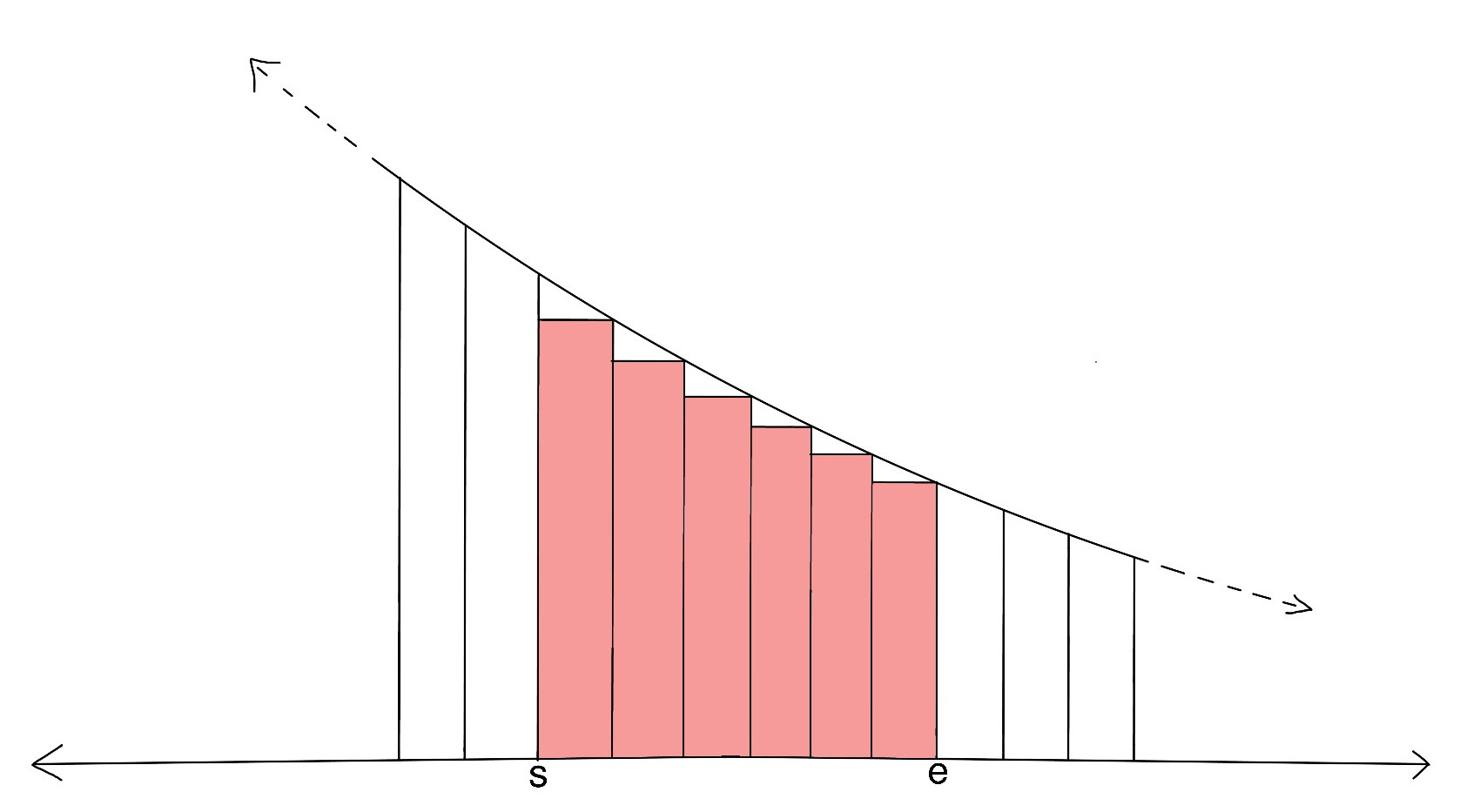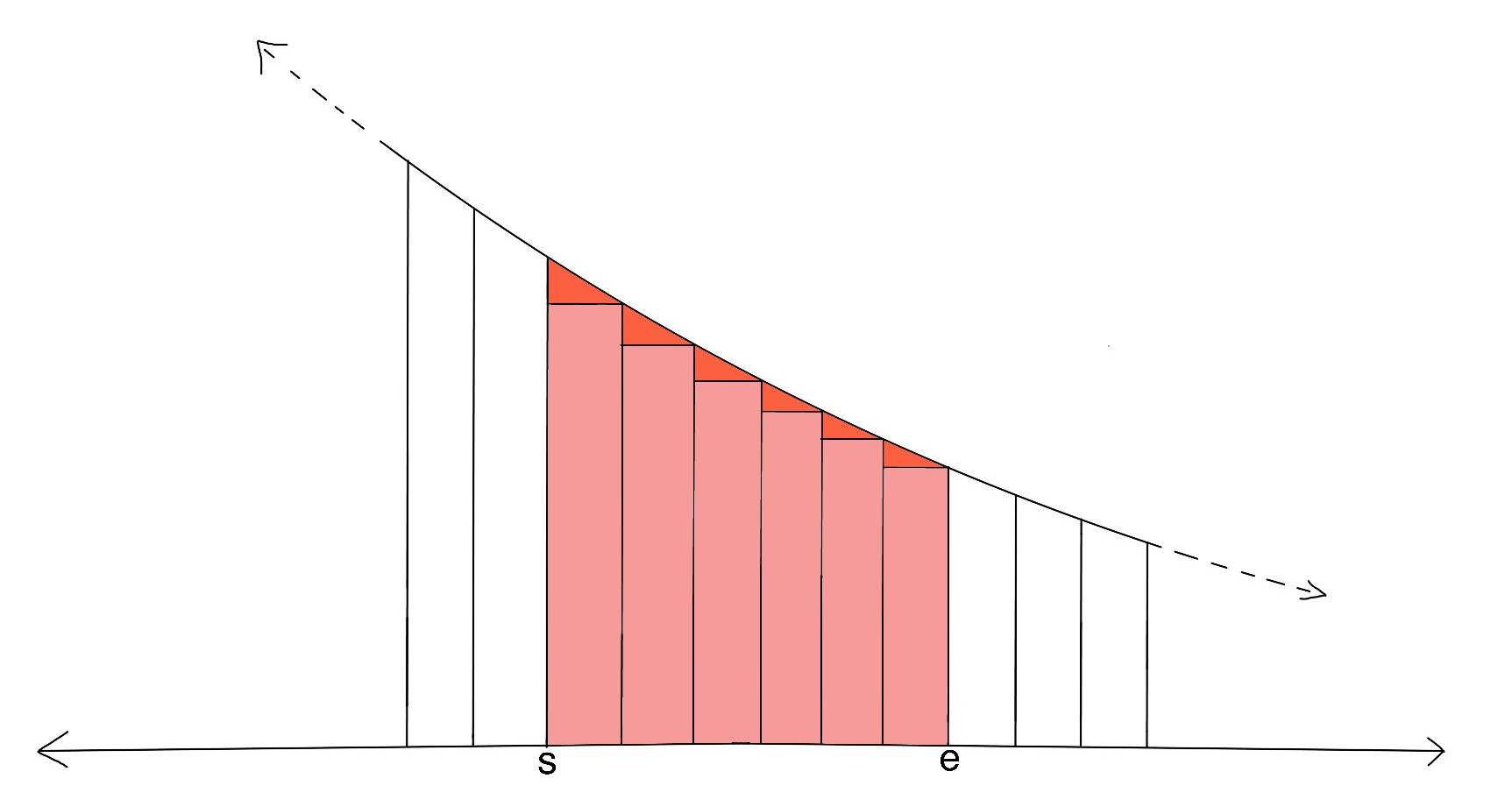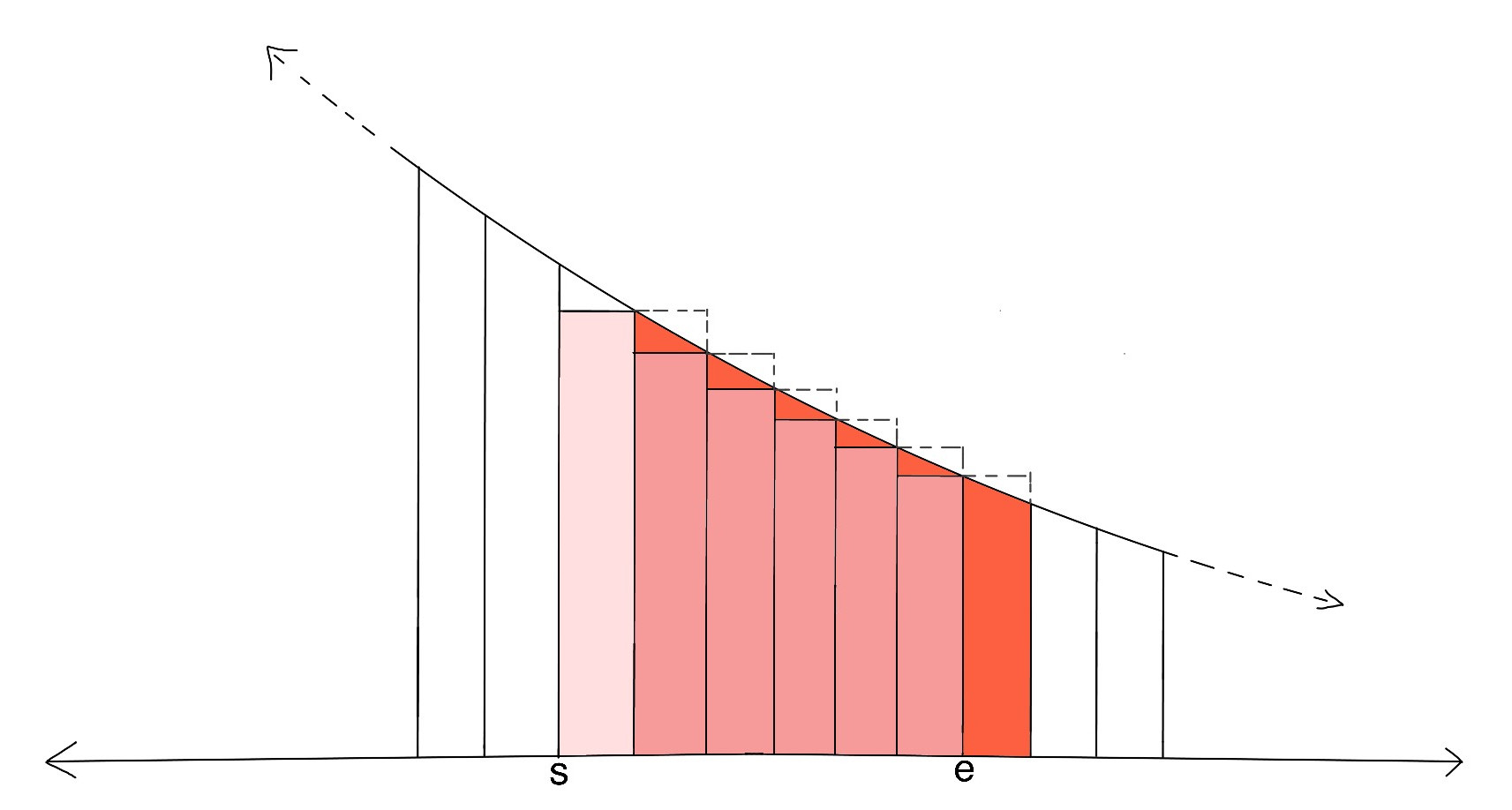The first image represents the actual number we need $$\sum_{x=s+1}^{e}\frac{1}{x}$$$, second image represents a larger area $$\int_{s}^{e}\frac{1}{x}\,dx$$$ and third image represents a smaller area $$\int_{s+1}^{e+1}\frac{1}{x}\,dx$$$. So, error (denoted as $$Er_{s,e}$$$) is given by:

$$Er_{s,e} \lt \int_{s}^{e}\frac{1}{x}\,dx - \int_{s+1}^{e+1}\frac{1}{x}\,dx$$$$$\Rightarrow Er_{s,e} \lt \int_{s}^{s+1}\frac{1}{x}\,dx - \int_{e}^{e+1}\frac{1}{x}\,dx \lt \int_{s}^{s+1}\frac{1}{x}\,dx$$$
$$\Rightarrow Er_{s,e} \lt \frac{1}{s}$$$Now, we are estimating only after $$10^6$$$, so $$s = 10^6$$$, hence $$Er_{10^6,e} \lt 10^{-6}$$$.

Test Data
info We recommend that you practice debugging solutions without looking at the test data.